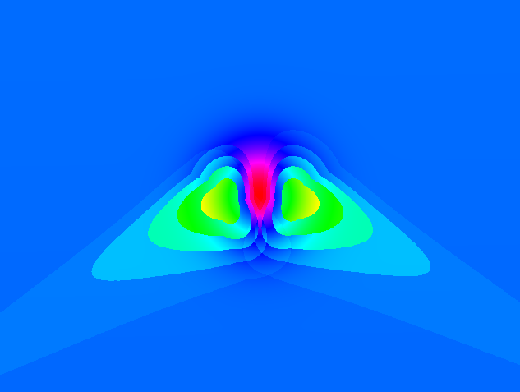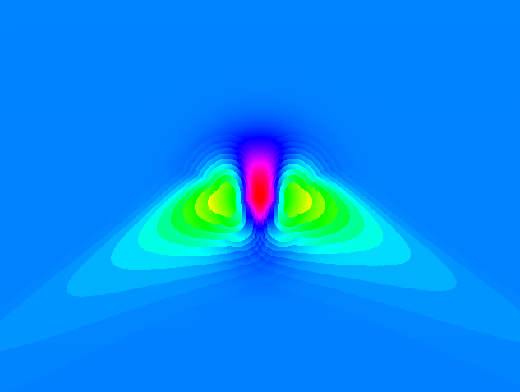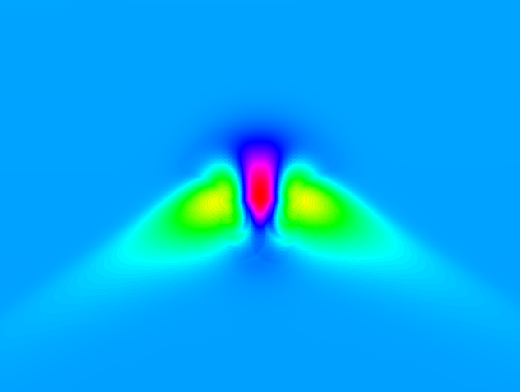R.sun

Tips

The speed of r.sun is much higher if r.horizon is used first and the resulting maps are given as input to r.sun. Background: the horizon needs to be computed only one time before, not in every step within r.sun. See the example at the end of the r.sun help page.

Testing

Create an artificial surface containing a Gaussian mound:

r.surf.volcano out=gauss method=gaussian kurtosis=1

Overlay some 100m contours to show underlying topography:

r.contour in=gauss out=gauss_100m_contours step=100
d.vect gauss_200m_contours color=white

Set map's color table to highlight detail:

Time step

The following three images demonstrate the effects of using different time step parameters.Default 30 minute time step over a Gaussian mound.15 minute time step over a Gaussian mound.